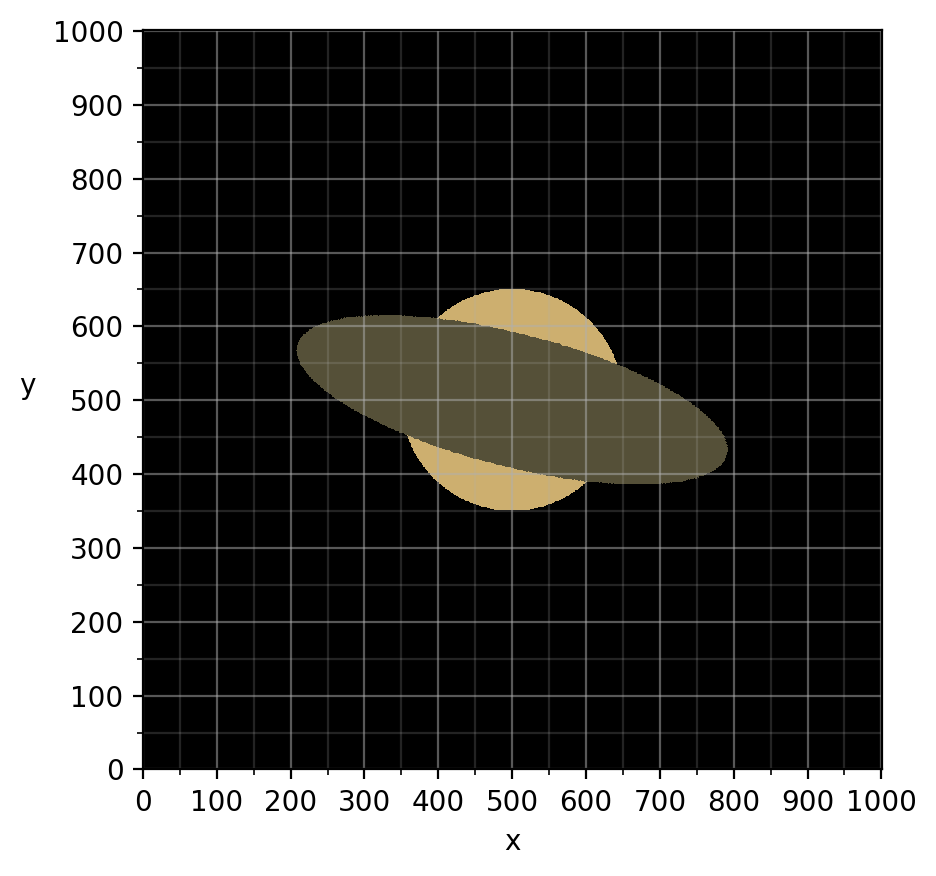# The full size canvas 🎨

Let's draw something a little more challenging on a larger scale.

We'll start by creating a 1000x1000 black canvas, the maximum allowed number of pixels (1 Megapixel).
Once it arrives, let's start drawing a planet, beginning with a nice large circle in the middle:

update bitmap_pixels set colour = case
when point (x,y) <@ circle '((500,500),150)' then '#CDAF6F'
else '#000000'
endNow let's draw a Saturn-like ring around it:

update bitmap_pixels set colour = case
when {{ is_in_oval(center=[500,500], diameter=600, ratio_x=1,ratio_y=0.3,rotation=0.25) }} then '#555038'
else colour
end

Hold up! What is this sorcery? {{ is_in_oval(..) }}?
That isn't valid SQL!

A seasoned dbt user will instantly recognise that this is Jinja, a Python-based templating language.
That's right, SQL Draw supports Jinja, and it's the key to moving your focus from low level pixel manipulation, to painting with a tool palette.
The is_in_oval macro is described here, which is a dbt docs page built off this git repository. Any Jinja macro in the main branch of this project (including any you provide to the Coalesce community via pull request) can be used in our SQL Draw commands. You can also define macros and write other Jinja statements directly in your SQL query.Anyway let's get back to our planet, we kinda botched it with that oval.
First, just type "undo" into the thread, this will undo the changes made by the last successful query.
Now we can try again, but this time we'll:

• Provide a series of rings instead of a solid oval
• Re-draw the circle first, but only where it's above the top half of the oval so that the rings appear to wrap behind it
In doing both of these, we'll lean on the fact that case statements are checked in order written, which provides a draw priority.

update bitmap_pixels set colour = case
-- y=mx+b formula, if above then render the circle first
when y > -0.25*x+600 and point (x,y) <@ circle '((500,500),150)' then '#CDAF6F'
when {{ is_in_oval(center=[500,500], diameter=400, ratio_x=1,ratio_y=0.3,rotation=0.25) }} then colour
when {{ is_in_oval(center=[500,500], diameter=430, ratio_x=1,ratio_y=0.3,rotation=0.25) }} then '#555038'
when {{ is_in_oval(center=[500,500], diameter=440, ratio_x=1,ratio_y=0.3,rotation=0.25) }} then colour
when {{ is_in_oval(center=[500,500], diameter=480, ratio_x=1,ratio_y=0.3,rotation=0.25) }} then '#F5C97E'
when {{ is_in_oval(center=[500,500], diameter=500, ratio_x=1,ratio_y=0.3,rotation=0.25) }} then colour
when {{ is_in_oval(center=[500,500], diameter=550, ratio_x=1,ratio_y=0.3,rotation=0.25) }} then '#C9A167'
when {{ is_in_oval(center=[500,500], diameter=590, ratio_x=1,ratio_y=0.3,rotation=0.25) }} then colour
when {{ is_in_oval(center=[500,500], diameter=660, ratio_x=1,ratio_y=0.3,rotation=0.25) }} then '#806144'
else colour
end

Much better!